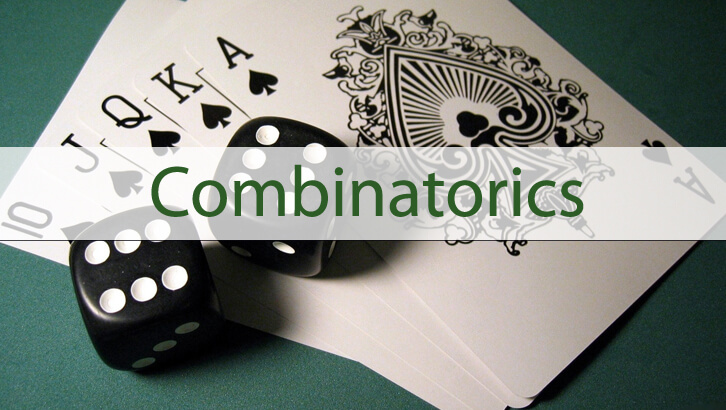# Quant Tips – Permutation and Combination – Fixing the Errors1. Five boys need to be allotted to 4 different rooms such that each boy is allotted a room and no room is empty. In how many ways can this be done?
Given solution
Let the boys be A, B, C, D and E. Let the rooms be 101, 102, 103 and 104. Now, we know that exactly one room will have two occupants. First, let us try to send 4 boys to 4 rooms, we can worry about the fifth occupant later on. Let us select 4 out of the 5 boys first. This can be done in 5C4 ways. Now, these 4 can be allotted to 4 different rooms in 4! ways. So, 4 boys in 4 rooms can be done in 5C4 * 4! = 5 * 24 = 120 ways.
Now, the fifth boy has to go into one of the rooms. He can do this in 4 ways as there are 4 different rooms available. So, total number of outcomes = 120 * 4 = 480.
Bug in the solution:
We end up double-counting here.
A, B, C and D could be allotted rooms 101, 102, 103 and 104 in that order. Post this, E could “double-up” with A. So, we would have A and E in 101, B in 102, C in 103 and D in 104.
In another scenario, E, B, C, D could be allotted 101, 102, 103 and 104 in that order. Post this, A could “double-up” with E. So, we would have A and E in 101, B in 102, C in 103 and D in 104.
The two scenarios mentioned above are identical. However, we end up counting both. This is the reason for the double count.
Note that in our method, we end up having a ‘first’ occupant for a room and a ‘second’ occupant. We say, A goes into room number 101 and then E ‘joins’ him. The moment you do that, ‘order’ creeps in. We end up factoring in order when we shouldn’t.

Awesome, isn’t it.

Correct solution:
The boys should be allotted into rooms as 2 + 1 + 1 + 1. As in, exactly one room has 2 occupants. Some two guys have to be room-mates. Let us first select these two room-mates. This can be done in 5C2 ways. After we have selected these two room-mates, we practically need to just allot these 4 ‘groups’ into 4 rooms. This can be done in 4! Ways.
Total number of options = 5C2 * 4! = 10 * 24 = 240 ways.
2. How many 4-digit numbers with 4 distinct digits are possible?
Given solution
Let the 4-digit number be ‘abcd’. Now,
‘d’ can take 10 possible values – 0 to 9.
‘c’ can take 9 possible values – 0 to 9 except d
‘b’ can take 8 possible values – 0 to 9 except d and c
‘a’ can take 6 possible values – 1 to 9 except d, b and c
So, total number of possibilities = 6 * 8 * 9 * 10 = 4320.
Bug in the solution:
The simple way of stating the bug is as follows “But one of b, c, d might have been zero”.
When we say a can take 6 possible values. We eliminate 0, b, c, and d from the list 0 to 9. If b, c or d were zero, then that in that case, a will still have 7 possible options.
In this question, the leading digit has a constraint. So, start with that. No point factoring in the constraint in the last step. Get that out of the way first.
Correct solution:
Let the 4-digit number be ‘abcd’. Now,
‘a’ can take 9 possible values – 1 to 9.
‘b’ can take 9 possible values – 0 to 9 except a
‘c’ can take 8 possible values – 0 to 9 except a and b
‘d’ can take 7 possible values – 1 to 9 except a, b and c
Total number of options = 9 * 9 * 8 * 7.
3. In how many ways can we rearrange the letters of the word TWOIIM such that the vowels appear together?
Given solution
Let us take the three vowels together and call it as X. Now, we need to rearrange the letters of the word TWMX. This can be done in 4! ways. Or, there are 24 ways of rearranging the letters of the word TWOIIM with vowels appearing together.
Bug in the solution:
We do not account for the different variations that ‘X’ can come in. Now, the three vowels have been replaced with X. Or, X is nothing but OII in some order. The key point here is that X could be OIO, IOO or OOI. X can take three different forms. The solution has overlooked this.
Correct solution:
Let us take the three vowels together and call it as X. Now, we need to rearrange the letters of the word TWMX. This can be done in 4! ways.
Now, the three vowels have been replaced with X. Or, X is nothing but OII in some order. The key point here is that X could be OIO, IOO or OOI. X can take three different forms.
So total number of possibilities = 4! * 3 = 72 ways.
_____
About the Author:
Rajesh Balasubramanian runs 2IIM’s CAT program and handles more than half the classes for CAT preparation. He completed his Electrical engineering from IIT Madras in 2001 and PGDM from IIM Bangalore in 2003. He worked as an equity Research Analyst at Credit Suisse, London. This was an enriching experience, in a literal sense; and a soul-sapping experience otherwise. He finally quit his job in 2009 and joined 2IIM as director in 2010.